# GED Math : Proportions and Percentages

## Example Questions

1 2 3 4 5 6 7 9 Next →

### Example Question #441 : Ged Math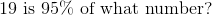Explanation:

The part, the whole, and the percent of a percentage problem can be related by the proportion statement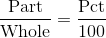Setequal to the whole, and 19 and 95 equal to the part and the percent, respectively: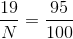Set the cross-products equal: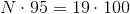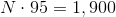Divide both sides by 95 to solve for: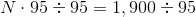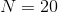,

the correct response.

### Example Question #442 : Ged Math

9 is what percent of 3?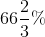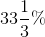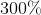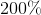Explanation:

If we let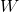andbe a part and a whole of a percent problem, then thethatis ofis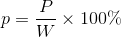.

In this problem, 9 is the part and 3 is the whole. Thus,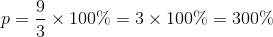,

the correct response.

### Example Question #443 : Ged Math

What is 9% of 3?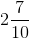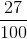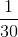Explanation: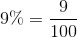, so 9% 0f 3 is equal to,

the correct response.

### Example Question #444 : Ged Math

In January, Sophia sold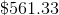worth of goods at her store. In February, she sold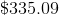worth of goods. What is the percent decrease from January to February?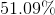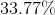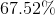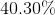Explanation:

Recall how to find the percent change for two values: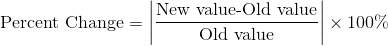The new value in this case would be the value from February, and the old value would be the value from January.

Plug in the correct values to find the percent change: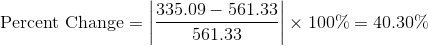### Example Question #445 : Ged Math

Among other things, a batch of cookies containscups of flower to everycups of oats and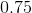cups of prune slices. If the batch contains a total ofcups, how many cups of prune slices are there in the batch?Explanation:

Based on the information provided, you know that a "standard" batch could be represented as:This lets you set up a proportion:The variablerepresents the number of cups of prunes in the full batch. Begin solving by cross-multiplying: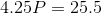Then, just divide by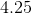:The entire recipe contains 6 cups of prune slices.

### Example Question #446 : Ged Math

In a sequence of numbers, the first value is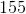.  Every number after that islarger than the one that comes before it.  What is theth element in the sequence?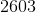Cannot be computed from the information provided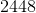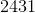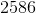Explanation:

To imagine your sequence, think of a few values: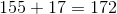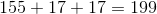And so forth...

In the second element, you add one value of seventeen. In the third, you add two. This means that in theth, you will add a total of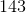seventeens; therefore, you could write your value as:1 2 3 4 5 6 7 9 Next →